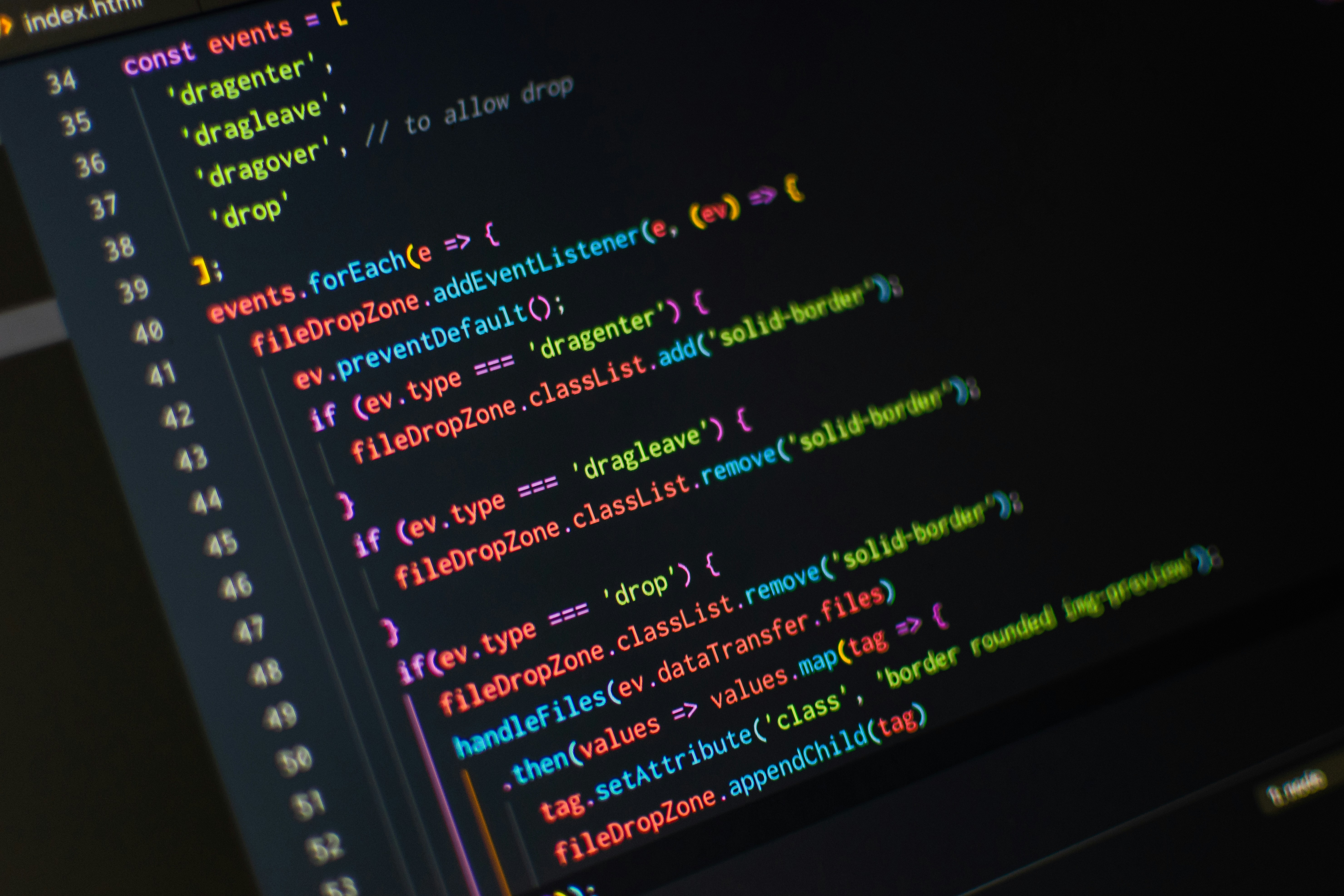# C++ 6. Basic ArithmeticIn part 5 you learned about memory concepts. In this article we will go through some basic arithmetic in C++. Table 1 below summarizes the C++ arithmetic operators.

 Operation C++ Operator Example Addition + x + y Subtraction - x - y Multiplication * x * y Division / x / y Modulus % x % y

Table 1

When you create a variable, you can actually use basic arithmetic like this:

``````int x = 10 + 10;
std::cout << x;``````

The compiler will first calculate 10 + 10, and then assign our variable the result of this calculation. Therefore, when we run this program it will output 20. In the same way, if we change our + sign to -, the output will be 0, since 10 - 10 is 0.

To perform multiplications, use the asterisk (*).

``````int x = 5 * 2;
std::cout << x;``````

This will output 10. If we use the same example with division, the output should be 2.5, true? False. Our variable x is declared as an integer, and integers only hold integers, any fractional part is discarded. No rounding occurs. To get the remainder after integer division, C++ provides the modulus operator (%). This operator can only be used with integer operands. Consider the code:

``````int x = 5 % 2;
std::cout << x;``````

The remainder of 5/2 is 1, so the output here will be 1.

The same rules of operator precedence that's in algebra hold in C++. This means that operators in expressions contained within pairs of parentheses are always evaluated first. Multiplication, division and modulus operations are applied next. These are applied from left to right if an expression contains several multiplication, division and modulus operations. Addition and subtraction are applied last, and are also applied from left to right.

In algebra it's common to put two variables next two each other when multiplying. This cannot be one in C++, every time we want to multiplicate two variables, we have to put the multiplication operator between them (*). Take the straight line as an example:

Algebra:

C++:

``y = m * x + b;``

In the next article we will learn about functions and namespace. Read C++ 7. Functions (and namespace) here.